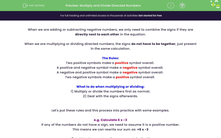# Multiply and Divide Directed Numbers

In this worksheet, students will practise multiplying and dividing positive and negative numbers, applying the rules related to directed numbers accurately.Key stage:  KS 4

Year:  GCSE

GCSE Subjects:   Maths

GCSE Boards:   Pearson Edexcel, OCR, Eduqas, AQA,

Curriculum topic:   Number, Number Operations and Integers

Curriculum subtopic:   Structure and Calculation Calculations with Integers

Difficulty level:#### Worksheet Overview

When we are adding or subtracting negative numbers, we only need to combine the signs if they are directly next to each other in the equation.

When we are multiplying or dividing directed numbers, the signs do not have to be together, just present in the same calculation.

The Rules:

Two positive symbols make a positive symbol overall.

A positive and negative symbol make a negative symbol overall.

A negative and positive symbol make a negative symbol overall.

Two negative symbols make a positive symbol overall.

What to do when multiplying or dividing:

1) Multiply or divide the numbers first as normal;

2) Deal with the signs afterwards.

Let's put these rules and this process into practice with some examples.

e.g. Calculate 6 x -3

If any of the numbers do not have a sign, we need to assume it is a positive number.

This means we can rewrite our sum as: +6 x -3

1) Multiply the numbers first as normal: 6 x 3 = 18

2) Deal with the signs afterwards: + and - makes a - overall

+6 x -3 = -18

e.g. Calculate -15 ÷ -5

1) Divide the numbers first as normal: 15 ÷ 5 = 3

2) Deal with the signs afterwards: - and - makes a + overall

15 ÷ 5 = +3

We don't put the sign with the answer if it's a positive number, so our answer should be: 15 ÷ 5 = 3

In this activity, you will practise multiplying and dividing positive and negative numbers, applying the rules related to directed numbers accurately.

You can always return to this page to review the rules if you need to at any point in this activity - good luck!

### What is EdPlace?

We're your National Curriculum aligned online education content provider helping each child succeed in English, maths and science from year 1 to GCSE. With an EdPlace account you’ll be able to track and measure progress, helping each child achieve their best. We build confidence and attainment by personalising each child’s learning at a level that suits them.

Get started